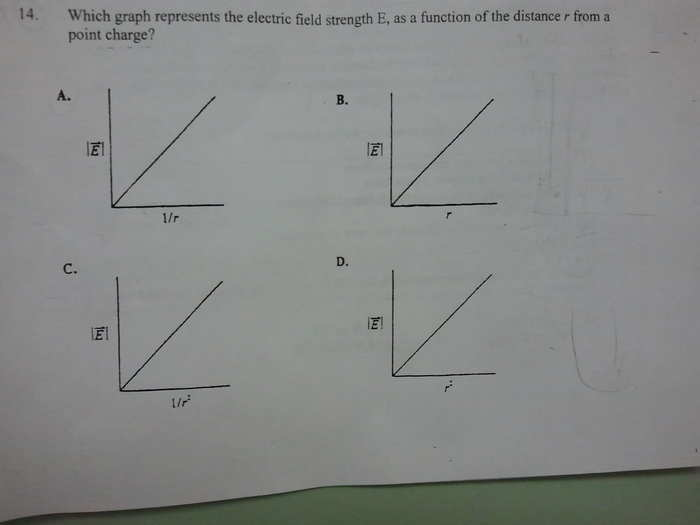# Relationship between Electric Fields and Distance from point charge?

Relationship between Electric Fields and Distance from point charge??

## Homework StatementE= kq/r^2

## The Attempt at a Solution

K so looking at the graph, I noticed that there is a inverse-square relationship between the electric field and the radius/distance.

So I considered that there would have to be a curved slope on a 1/r^2 graph, because the x-axis is squared.

Wouldn't any graph have a curved slope when the x-axis is squared?

For example, a simple equation like y = x^2 results in a curved slope, because the y-axis point is always squared of the x-axis point.

Wouldn't that reasoning work with this?

But my friend is saying that the graph would be curved with just 1/r.

And straight with 1/r^2. So her answer is C.

Which is right? Other ppl in my class are also saying that the answer is C as well, but i dont see why.

A lot of people are saying I'm thinking too hard and reaching a wrong conclusion, but they don't know how to explain.

What's wrong with my theory and what's right about my friend's?

## The Attempt at a Solution

SammyS
Staff Emeritus
Homework Helper
Gold Member

What would the markings look like on the horizontal axis of the 1/r2 graph ?

What would they look like on the 1/r graph?

What would the markings look like on the horizontal axis of the 1/r2 graph ?

What would they look like on the 1/r graph?

On the 1/r^2 graph, markings on the x-axis would go

1, 1/4, 1/9, etc

On the 1/r graph, markings would go

1, 1/2, 1/3, etc

This... Does not help me get it.